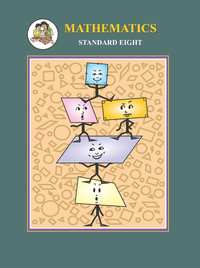SSC (English Medium) Class 8Maharashtra State Board
Share

# Balbharati solutions for Class 8 Mathematics chapter 5 - Expansion formulae

#### Balbharati Balbharati Class 8 Mathematics## Chapter 5: Expansion formulae

Practice Set 5.1Practice Set 5.2Practice Set 5.3Practice set 5.3Practice Set 5.4

#### Chapter 5: Expansion formulae Exercise Practice Set 5.1 solutions [Page 24]

Practice Set 5.1 | Q 1 | Page 24

Expand.

(a+2)(a−1)

Practice Set 5.1 | Q 2 | Page 24

Expand.

(m−4)(m+6)

Practice Set 5.1 | Q 3 | Page 24

Expand.

(p+8) (p−3)

Practice Set 5.1 | Q 4 | Page 24

Expand.

(13+x) (13−x)

Practice Set 5.1 | Q 5 | Page 24

Expand.

(3x+4y) (3x+5y)

Practice Set 5.1 | Q 6 | Page 24

Expand.

(9x−5t) (9x+3t)

Practice Set 5.1 | Q 7 | Page 24

Expand.

(m+2/3)(m-7/3)

Practice Set 5.1 | Q 8 | Page 24

Expand.

(x+1/x)(x-1/x)

Practice Set 5.1 | Q 9 | Page 24

Expand.

(1/y+4)(1/y-9)

#### Chapter 5: Expansion formulae Exercise Practice Set 5.2 solutions [Page 25]

Practice Set 5.2 | Q 1 | Page 25

Expand

(k + 4)3

Practice Set 5.2 | Q 2 | Page 25

Expand

(7x + 8y)3

Practice Set 5.2 | Q 3 | Page 25

Expand

(7 + m)3

Practice Set 5.2 | Q 4 | Page 25

Expand

(52)3

Practice Set 5.2 | Q 5 | Page 25

Expand

(101)3

Practice Set 5.2 | Q 6 | Page 25

Expand

(x + 1/x)^3

Practice Set 5.2 | Q 7 | Page 25

Expand

(2m + 1/5)^3

Practice Set 5.2 | Q 8 | Page 25

Expand

((5x)/y + y/(5x))^3

#### Chapter 5: Expansion formulae Exercise Practice Set 5.3, Practice set 5.3 solutions [Page 27]

Practice Set 5.3 | Q 1.1 | Page 27

Expand

(2m − 5)3

Practice Set 5.3 | Q 1.2 | Page 27

Expand

(4 − p)

Practice Set 5.3 | Q 1.3 | Page 27

Expand

(7x − 9y)3

Practice Set 5.3 | Q 1.4 | Page 27

Expand

(58)3

Practice Set 5.3 | Q 1.5 | Page 27

Expand

(198)3

Practice Set 5.3 | Q 1.6 | Page 27

Expand

(2p - 1/(2p))^3

Practice Set 5.3 | Q 1.7 | Page 27

Expand

(1 - 1/a)^3

Practice Set 5.3 | Q 1.8 | Page 27

Expand

(x/3 - 3/x)^3

Practice Set 5.3 | Q 2.1 | Page 27

Simplify

(2a + b)− (2a − b)3

Practice Set 5.3 | Q 2.2 | Page 27

Simplify

(3r − 2k)3 + (3r + 2k)3

Practice Set 5.3 | Q 2.3 | Page 27

Simplify

(4a − 3)− (4a +3)3

Practice set 5.3 | Q 2.4 | Page 27

Simplify

(5x − 7y)+ (5x + 7y)3

#### Chapter 5: Expansion formulae Exercise Practice Set 5.4 solutions [Page 28]

Practice Set 5.4 | Q 1.1 | Page 28

Expand.

(2p + q +5)2

Practice Set 5.4 | Q 1.2 | Page 28

Expand.

(m + 2n +3r)2

Practice Set 5.4 | Q 1.3 | Page 28

Expand.

(3x +4y − 5p)2

Practice Set 5.4 | Q 1.4 | Page 28

Expand.

(7m − 3n − 4k)2

Practice Set 5.4 | Q 2.1 | Page 28

Simplify.

(x − 2y +3)2 + (x + 2y − 3)2

Practice Set 5.4 | Q 2.3 | Page 28

Simplify.

(3k − 4r − 2m)− (3k + 4r − 2m)2

Practice Set 5.4 | Q 2.3 | Page 28

Simplify.

(7a − 6b + 5c)+ (7a + 6b − 5c)2

## Chapter 5: Expansion formulae

Practice Set 5.1Practice Set 5.2Practice Set 5.3Practice set 5.3Practice Set 5.4

#### Balbharati Balbharati Class 8 Mathematics## Balbharati solutions for Class 8 Mathematics chapter 5 - Expansion formulae

Balbharati solutions for Class 8 chapter 5 (Expansion formulae) include all questions with solution and detail explanation. This will clear students doubts about any question and improve application skills while preparing for board exams. The detailed, step-by-step solutions will help you understand the concepts better and clear your confusions, if any. Shaalaa.com has the Maharashtra State Board Balbharati Class 8 Mathematics solutions in a manner that help students grasp basic concepts better and faster.

Further, we at Shaalaa.com provide such solutions so that students can prepare for written exams. Balbharati textbook solutions can be a core help for self-study and acts as a perfect self-help guidance for students.

Concepts covered in Class 8 Mathematics chapter 5 Expansion formulae are Expansion of (X + A) (X + B), Expansion of (A + B)3, Expansion of (A - B)3, Expansion of (A + B + C)2.

Using Balbharati Class 8 solutions Expansion formulae exercise by students are an easy way to prepare for the exams, as they involve solutions arranged chapter-wise also page wise. The questions involved in Balbharati Solutions are important questions that can be asked in the final exam. Maximum students of Maharashtra State Board Class 8 prefer Balbharati Textbook Solutions to score more in exam.

Get the free view of chapter 5 Expansion formulae Class 8 extra questions for and can use Shaalaa.com to keep it handy for your exam preparation

S